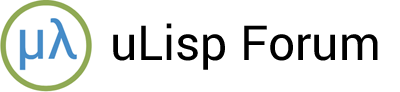# Printing a float with a certain number of digits after the decimal point

#1

Hi

Is it possible to print floats with a specified number of digits after the decimal point ?
Suppose number is 3.1425826 and I want only the 2 numbers after the decimal point
so it would print 3.14.
Could this be done using calculations , or another way ?

Thanks Ronny Suy

#2

A simple way would be to convert the floating-point number to a string:

``````> (princ-to-string (/ 9 7))
"1.28571"
``````

then use subseq to extract the part you want:

``````> (subseq (princ-to-string (/ 9 7)) 0 4)
"1.28"
``````

Here’s a slightly better solution that rounds correctly:

``````(defun dp2 (x)
(let* ((int (truncate x))
(dp (round (* 100 (- x int)))))
(princ int) (princ #\.) (princ dp))
nothing)
``````

This gives:

``````>  (dp2 (/ 9 7))
1.29``````